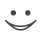# This flashcard is just one of a free flashcard set. See all flashcards!

109
Which of the following describes tailing the hedge?
A.A strategy where the hedge position is increased at the end of the life of the hedge
B.A strategy where the hedge position is increased at the end of the life of the futures contract
C.A more exact calculation of the hedge ratio when forward contracts are used for hedging
D.None of the above